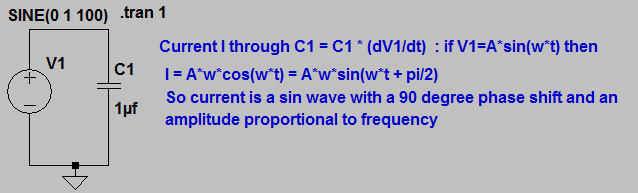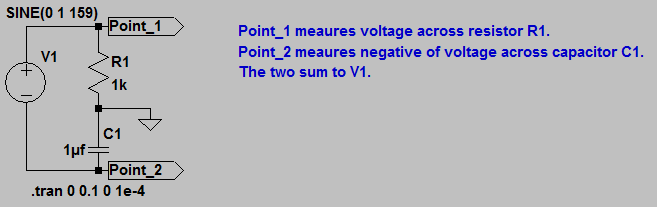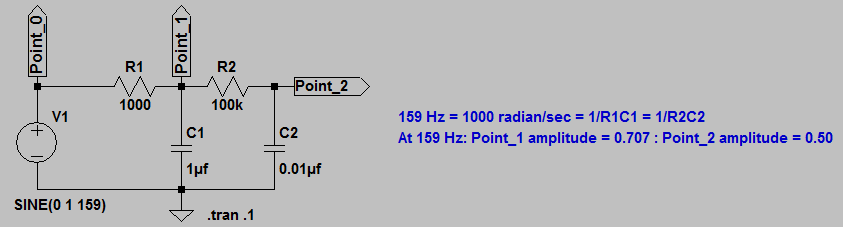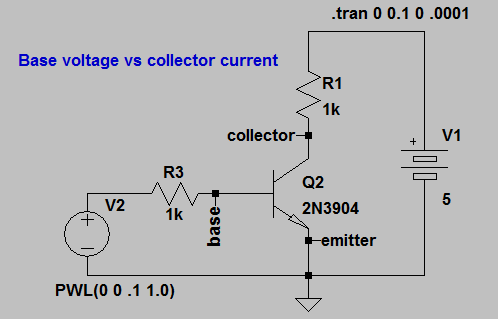## ECE 1810 (ENGRI 1810) Homework 2

1. Use LTspice to simulate the circuit shown.1. Vary the frequency of the sin wave source and show that peak current is proportional to frequency.
2. Vary the amplitude of the sin wave source and show that peak current is proportional to voltage amplitude.
3. Meaure the phase shift between current and votage at several frequencies. Is it always 90 degrees?
2. Use TSpice to explore the circuit shown.1. Using a sin wave source show that the voltage across the resistor and across the capacitor are always 90 degrees out of phase.
2. Add the voltages at Porint_1 and Point_2 and verify that they equal V1. Do this by clicking in the waveform window, then using the menu item `PlotSettings>Add Trace`. In the dialog box click on `V[point_1]`, type '+' then click on `V[point_2]` and click `OK`.
3. Use TSpice to explore the circuit shown.1. Using a sin wave source plot the amplitude at point_1 against frequency.
What happens to the phase of the sin wave?
2. Using a pulse source, measure the RC time constant R1C1 from the simulated waveform at point_1.
3. A slightly modified version allows you to get the amplitude and phase at all frequencys in one simulation. What is the relationship between the phase at Point_1 and Point_2 at all freqeucies?Run the simulation, and plot Vbase and current through R1. Make sure the waveform window is active, then using the menu item `PlotSettings>Add Trace`. In the dialog box click on `Ic(Q2)`,then edit to` log(Ic(Q2))` and click `OK`. If the following equation is correct then you should get a straight line. Do you? If not over what current range does the relationship work?